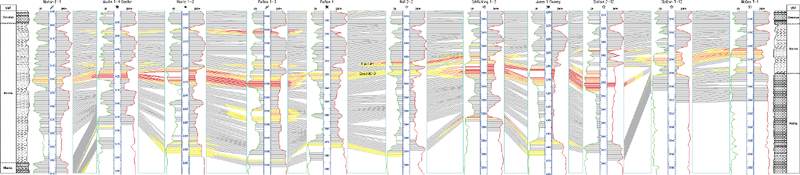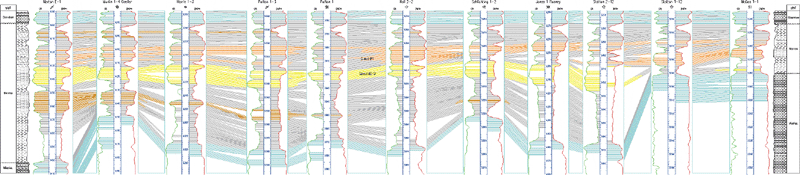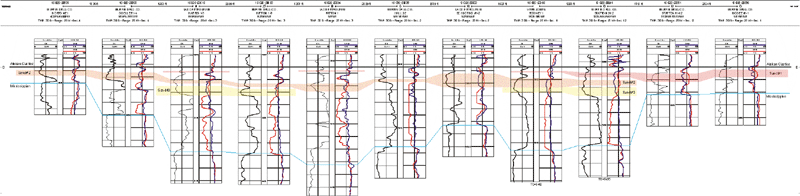## Cross-sections: Autocorrelation and Integrated

Original (1980’s) correlation defined the Morrow as a single interval based solely on gamma ray identification of sandstone and porosity cut-off to define net pay. Autocorrelation (concepts explained to right), utilizing a pattern-matching scheme using log response, provided correlation for three layers defined initially in the Harris #1-3 well. Integrating core analysis, the bottom correlation was constructed utilizing interpretation of log response (i.e., manual pattern-matching) filtered through constraints imposed by a depositional model.

Both recent modeling approaches accurately define the base of the Morrow Sandstone and the principal pay sand #2. The autocorrelation model presents a different interpretation of correlation for sand #1 due to lithologic complexity introduced by greater argillaceousness and due to lack of information concerning a depositional model.

Autocorrelation cross-sections based on field-wide correlation

## 3-D Autocorrelation

Geological correlations of wireline logs from wells along the computer-generated cross sections were made using the computer program CORRELATOR (Olea 1988). The program searches for correlations by comparing pairs of sequences of measurements, typically wireline logs from vertical wells. CORRELATOR usually processes two log traces per well, referred to as a "shale log" and a "correlation log." In this study, the shale log is a gamma ray log and the correlation log the average of a density log and a neutron log.

Let us call "reference well" the well on the left side of a display and "matching well" to the well to the right. At any given depth zi in the reference well, CORRELATOR automatically selects the depth zkbest in the matching well that maximizes the weighted correlation coefficient as the correlated position. If the log traces are sampled at regular intervals, the weighted correlation coefficient w1,2,3,4(i,k;n) is defined as the product of the standardized shale similarity coefficient a1,3(i,k;n) for the shale logs and the Pearsonian correlation coefficient r2,4(i,k;n) for the correlation logs: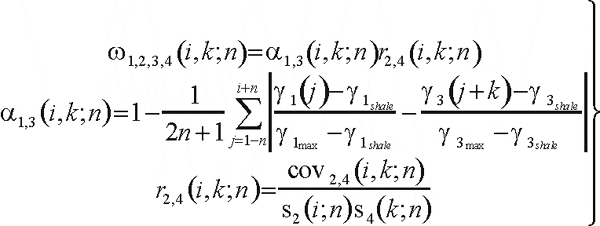The standardized shale similarity, the covariance cov2,4(i,k;n), and the standard deviations s2(i;n) and s4(k;n), are each calculated over correlation intervals of 2n+1 readings. The correlation coefficient reflects the degree of resemblance in shape of the log traces within the intervals of the two wells being compared. The shale similarity is sensitive to the difference in the relative proportion of shale within the intervals in the two wells. Maximizing the weighted correlation coefficient requires the specification of an additional parameter, the interval kmin<k<kmax over which the search for the best match will be made. The automatic maximization of the weighted correlation coefficient always yields one largest value w1,2,3,4max(i,kbest;n) for every elevation zi in the reference well. However, not all of these best matches, or tentative stratigraphic correlations, are retained. In an initial step, the system discards all weak correlations that are below a specified threshold value. In a later step, an expert system algorithm checks for global consistency of correlations and identifies any lines of correlation that cross other lines of correlation or that deviate significantly from the pattern formed by the correlations between other intervals. The expert system interactively displays these suspect correlations and suggests why they may be erroneous.

CORRELATOR first compares two wells and stores the acceptable correlations in a file. Then, a third well is compared to one of the original wells and the process repeated. By successively adding wells to the end of the growing cross section, a file of correlations is constructed that extends the length of the line of wells. The poster shows two modes of display. In the first one, given key elevations at one well, the program finds the equivalent elevations in all the wells of a cross-section. In the second display, values of gamma ray and average porosity, fla(i), are combined to produce a value of porosity corrected by shale effect, flc(i), which are used to generate a false-color display: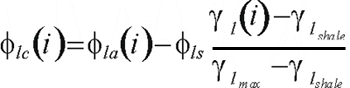(Click below for larger Image)